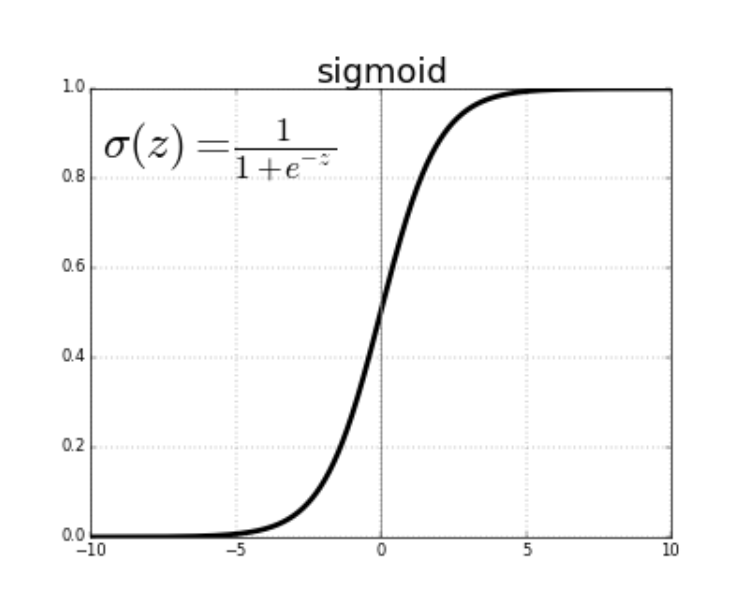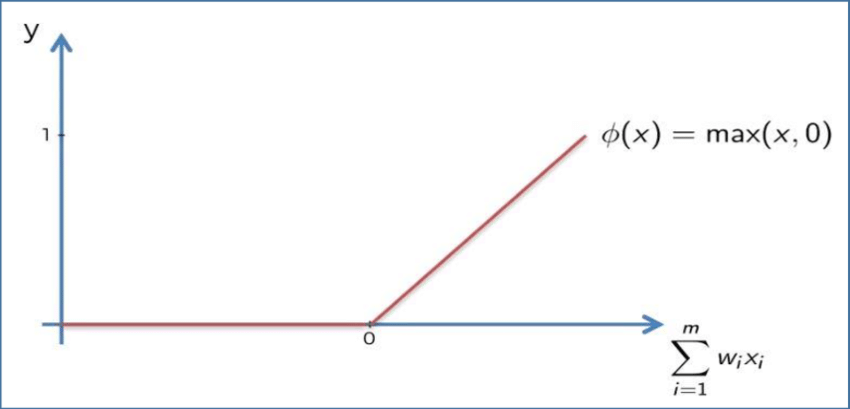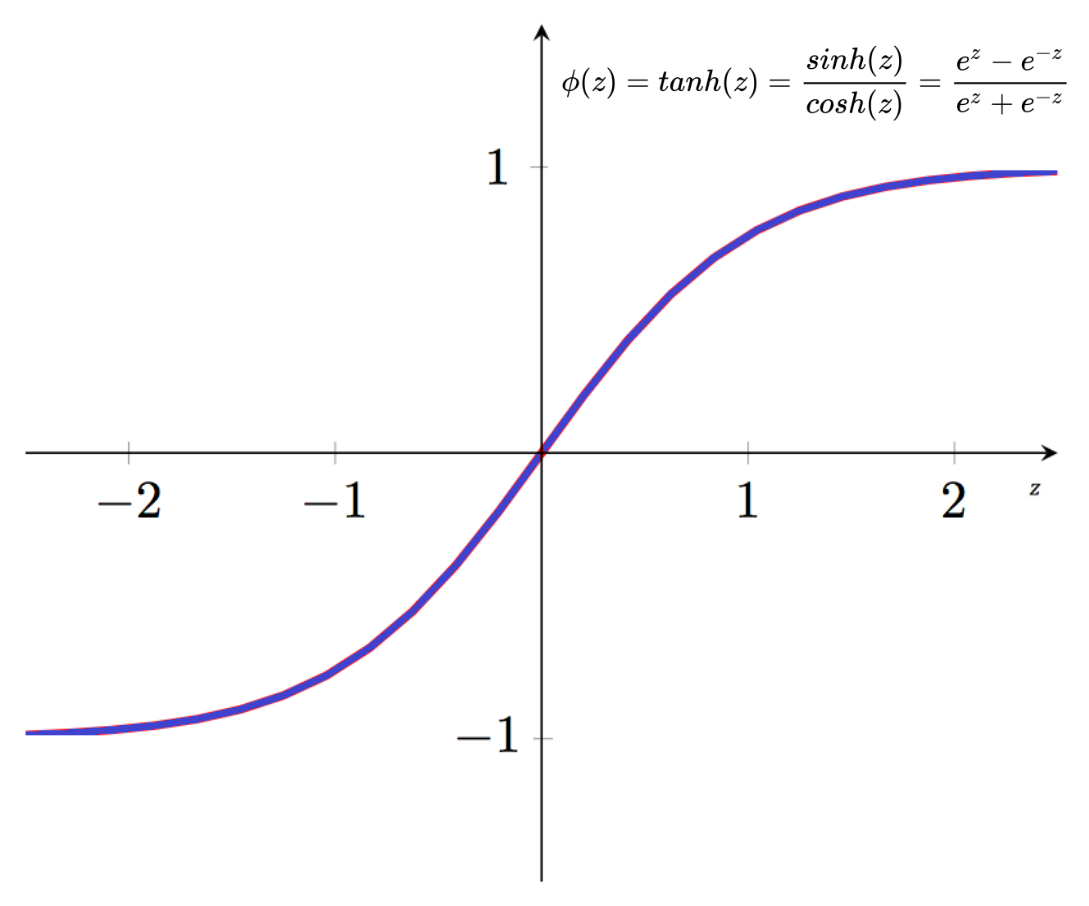# Deep Learning Activation Functions

Hey - Nick here! This page is a free excerpt from my \$199 course Python for Finance, which is 50% off for the next 50 students.

If you want the full course, click here to sign up.

Activation functions are a core concept to understand in deep learning.

They are what allows neurons in a neural network to communicate with each other through their synapse.

In this tutorial, you will learn to understand the importance and functionality of activation functions in deep learning.

## Table of Contents

You can skip to a specific section of this deep learning activation function tutorial using the table of contents below:

## What Are Activation Functions in Deep Learning?

In the last section, we learned that neurons receive input signals from the preceding layer of a neural network. A weighted sum of these signals is fed into the neuron's activation function, then the activation function's output is passed onto the next layer of the network.

There are four main types of activation functions that we'll discuss in this tutorial:

• Threshold functions
• Sigmoid functions
• Rectifier functions, or ReLUs
• Hyperbolic Tangent functions

Let's work through these activations functions one-by-one.

## Threshold Functions

Threshold functions compute a different output signal depending on weather or not its input lies above or below a certain threshold. Remember, the input value to an activation function is the weighted sum of the input values from the preceding layer in the neural network.

Mathematically speaking, here is the formal definition of a deep learning threshold function:As the image above suggests, the threshold function is sometimes also called a `unit step function`.

Threshold functions are similar to boolean variables in computer programming. Their computed value is either `1` (similar to `True`) or `0` (equivalent to `False`).

## The Sigmoid Function

The sigmoid function is well-known among the data science community because of its use in logistic regression, one of the core machine learning techniques used to solve classification problems.

The sigmoid function can accept any value, but always computes a value between `0` and `1`.

Here is the mathematical definition of the sigmoid function:One benefit of the sigmoid function over the threshold function is that its curve is smooth. This means it is possible to calculate derivatives at any point along the curve.

## The Rectifier Function

The rectifier function does not have the same smoothness property as the sigmoid function from the last section. However, it is still very popular in the field of deep learning.

The rectifier function is defined as follows:

• If the input value is less than `0`, then the function outputs `0`
• If not, the function outputs its input value

Here is this concept explained mathematically:Rectifier functions are often called `Rectified Linear Unit` activation functions, or `ReLUs` for short.

## The Hyperbolic Tangent Function

The hyperbolic tangent function is the only activation function included in this tutorial that is based on a trigonometric identity.

It's mathematical definition is below:The hyperbolic tangent function is similar in appearance to the sigmoid function, but its output values are all shifted downwards.

## Final Thoughts

In this tutorial, you had your first exposure to activation functions in deep learning. Although it may not yet be clear when we would use a specific function, this will become more clear as you work through this course.

Here is a brief summary of what you learned in this section:

• How activation functions are used in neural networks
• The definitions of threshold functions, sigmoid functions, rectifier functions (or ReLUs), and hyperbolic tangent functions